## 基于能量密度等效的超弹性压入模型与双压试验方法 1)

*西南交通大学力学与工程学院应用力学与结构安全四川省重点实验室, 成都 610031

## HYPERELASTIC INDENTATION MODELS AND THE DUAL-INDENTATION METHOD BASED ON ENERGY DENSITY EQUIVALENCE 1)

Zhang Xirun*, Cai Lixun,*,2), Chen Hui

*Applied Mechanics and Structure Safety Key Laboratory of Sichuan Province, School of Mechanics and Engineering, Southwest Jiaotong University, Chengdu 610031, China

School of Architecture and Construction, Changsha University of Science and Technology, Changsha 410114, China

 基金资助: 1)国家自然科学基金.  11872320

Received: 2020-01-15   Accepted: 2020-03-6   Online: 2020-05-18Abstract

For the hyperelastic problems of materials under indentation conditions, based on the mean-value energy density equivalence principle, semi-theoretical hyperelastic-material indentation models(SHIM) are proposed to describe the relationship among load, depth, indenter dimension and Mooney-Rivlin constitutive parameters under independent indentation with spherical indenter, flat indenter and conical indenter, respectively, and then the indentation method due to dual indenters(IMDI) is presented. The forward verification shows that, based on a series constitutive relation parameters of hyperelastic materials, the force-depth curves of spherical, conical and flat indentation respectively predicted by SHIM are closely consistent with the FEA results; and the reverse verification shows that, based on the force-depth curves under FEA conditional constitutive relation of a series of hyperelastic materials, the Mooney-Rivlin constitutive relations predicted by the dual indentation experimental method are closely consistent with the FEA conditional constitutive relations. For three hyperelastic rubbers, the spherical, flat and conical indentation tests were carried out, three constitutive relationships of the hyperelastic rubbers obtained by IMDI are all in good agreement with the uniaxial tensile results.

Keywords： hyperelastic material ; energy density equivalence ; semi-analytical ; indentation test ; constitutive relationship ; test method

Zhang Xirun, Cai Lixun, Chen Hui. HYPERELASTIC INDENTATION MODELS AND THE DUAL-INDENTATION METHOD BASED ON ENERGY DENSITY EQUIVALENCE 1). Chinese Journal of Theoretical and Applied Mechanics[J], 2020, 52(3): 787-796 DOI:10.6052/0459-1879-20-023

## 引言

1940年代初, Treloar[4-5]通过构造三链分子网络模型, 提出了表征应变能密度的Neo-Hookean模型, 该模型对橡胶拉伸应力-伸长比曲线的初始线性段有较为准确的描述, 而因采用单参数, 模型对曲线后继非线性段描述性较差. 1940年, Mooney考虑代表性体积单元(representative volume element, RVE). RVE是连续介质力学的基本六面体单元, 只要取合适的尺寸, 就能用以求解受载构元的宏观力学行为)应变能密度与3个主伸长比$\lambda_{i}$ $(i=1,2,3)$之间的对称关系, 提出了双参数超弹性材料本构关系. 1948年, Rivlin利用材料RVE变形张量不变量, 根据材料体积不可压缩性, 令第三不变量$I_{3}=\lambda_{1}^{2}\lambda_{2}^{2}\lambda_{3}^{2}=1$, 则Mooney本构方程可简化为连续介质力学框架下关于第一不变量$I_{1}$和第二不变量$I_{2}$的双参数方程形式

$\begin{equation} \label{eq1} \left. {\begin{array}{l} u=C_{10} (I_1 -3)+C_{01} (I_2 -3) \\ \left. {\begin{array}{l} I_1 =\sum_{i=1}^3 {\lambda _i^2 } \\ I_2 =\sum\limits_{\stackrel{i,j=1}{i\ne j}}^3\lambda _i^2 \lambda _j^2 \\ \lambda _i ={e}^{\varepsilon _i},\ \ i = 1,2,3 \\ \end{array}} \right. \\ \end{array}} \right\} \end{equation}$

## 1 半解析超弹性压入模型SHIM

### 1.1 Mooney-Rivlin模型的应力应变形式

$\begin{equation} \label{eq2} \sigma _{ij} =\frac{\partial u}{\partial \varepsilon _{ij} }=\frac{\partial u}{\partial I_1 }\frac{\partial I_1 }{\partial \varepsilon _{ij} }+\frac{\partial u}{\partial I_2 }\frac{\partial I_2 }{\partial \varepsilon _{ij} }+\frac{\partial u}{\partial I_3 }\frac{\partial I_3 }{\partial \varepsilon _{ij} } \end{equation}$

$\tau _i =2\left( {\lambda _i^2 \frac{\partial u}{\partial I_1 }-\lambda _i^{-2} \frac{\partial u}{\partial I_2 }} \right)+P,\ \ i=1, 2,3$

$\tau _1 =2\left( {\lambda _1^2 -\lambda _1^{-1} } \right)\left( {\frac{\partial u}{\partial I_1 }+\lambda _1^{-1} \frac{\partial u}{\partial I_2 }} \right)$

$\tau _1 =2\left( {\lambda _1^2 -\lambda _1^{-1} } \right)\left( {C_{10} +\lambda _1^{-1} C_{01} } \right)$

$\sigma _{g} =2\left( {\lambda -\lambda ^{-2}} \right)\left( {C_{10} +\lambda ^{-1}C_{01} } \right)$

### 1.2 基于能量密度等效方法的半解析压入模型

$u_{M} =\frac{U}{V_{{eff}} }=\frac{\iiint_\varOmega {u(x,y,z){d}V}}{V_{{eff}} }$

$u_{M} =C_{10} (I_1 -3)+C_{01} (I_2 -3)$

$U=u_{M} V_{{eff}} =\left[ {C_{10} (I_1 -3)+C_{01} (I_2 -3)} \right]V_{{eff}}$

$\begin{equation} \label{eq10} \left. {\begin{array}{l} U=\left( {C_{10} f_1 +C_{01} f_2 } \right)D^3 \\ \left. {\begin{array}{l} f_1 =(I_1 -3)\dfrac{V_{{eff}} }{D^3} f_2 =(I_2 -3)\dfrac{V_{{eff}} }{D^3} \\ \end{array}} \right. \\ \end{array}} \right\} \end{equation}$

$\begin{equation} \label{eq11} \left. {\begin{array}{l} f_1 =k_1 \lt(\dfrac{h}{D})^{k_0 } \\ f_2 =k_2 \lt(\dfrac{h}{D})^{k_0 } \\ \end{array}} \right\} \end{equation}$

$U=(k_1 C_{10} +k_2 C_{01} )D^3\lt(\frac{h}{D})^{k_0 }$

$U=W=\int {P{d}h}$

$\begin{equation} \label{eq14} \left. {\begin{array}{l} P=P^* \left( {\dfrac{h}{D}} \right)^{k_0 -1} \\ \left. {\begin{array}{l} P^* =CD^2 \\ C=k_0 (k_1 C_{10} +k_2 C_{01} ) \\ \end{array}} \right. \\ \end{array}} \right\} \end{equation}$

$\begin{equation} \label{eq15} \left. {\begin{array}{l} C=k_0 (k_1 C_{10} +k_2 C_{01} ) \\ {C}'={k}'_0 ({k}'_1 C_{10} +{k}'_2 C_{01} ) \\ \end{array}} \right\} \end{equation}$

### 图1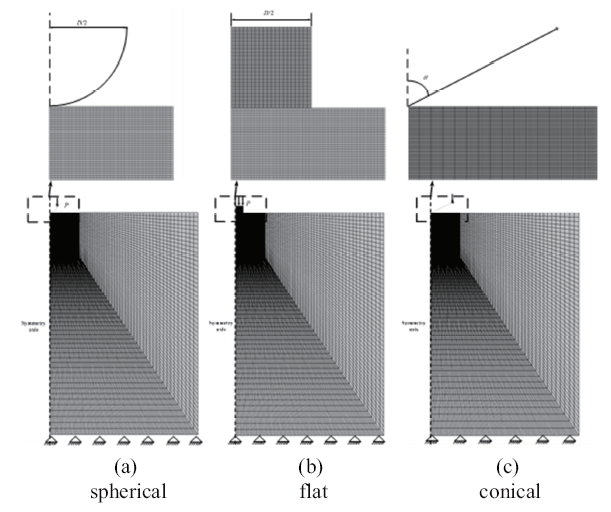Fig.1   The axisymmetric FEA indentation model

### 图2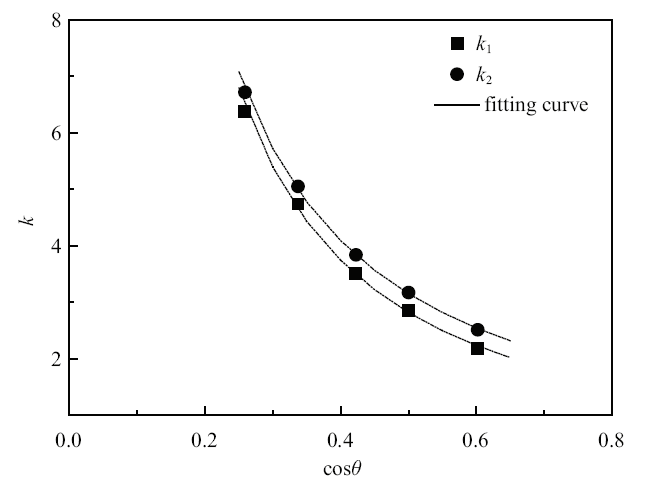Fig.2   Variations of deformation coefficient $k_{1}$, $k_{2}$ with cos$\theta$

Table 1  Parameters of SHIM for spherical and flat indentation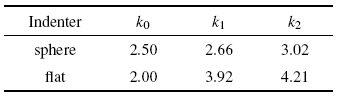Table 2  Parameters of SHIM for conical indentation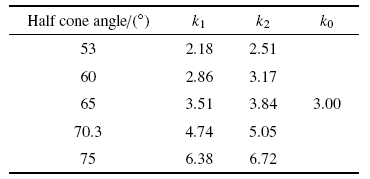$k_{i,i=1,2} =\beta _{i1} (\cos \theta )^{\beta _{i2} }$

Table 3  Deformation coefficient $k$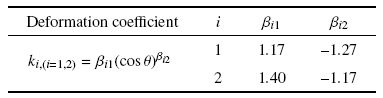## 2 有限元验证

### 图3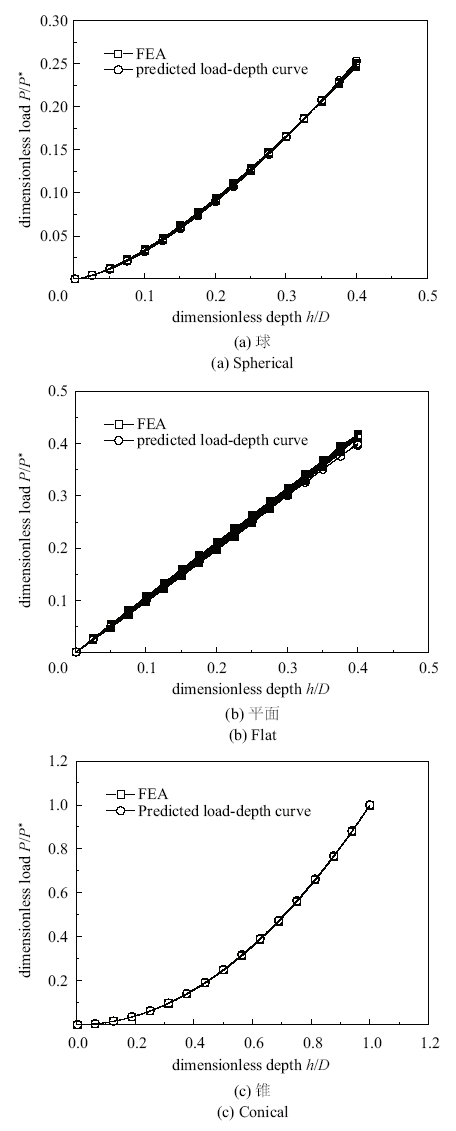Fig.3   Comparisons of predicted $P$-$h$ indentation curves and those from FEA

### 图4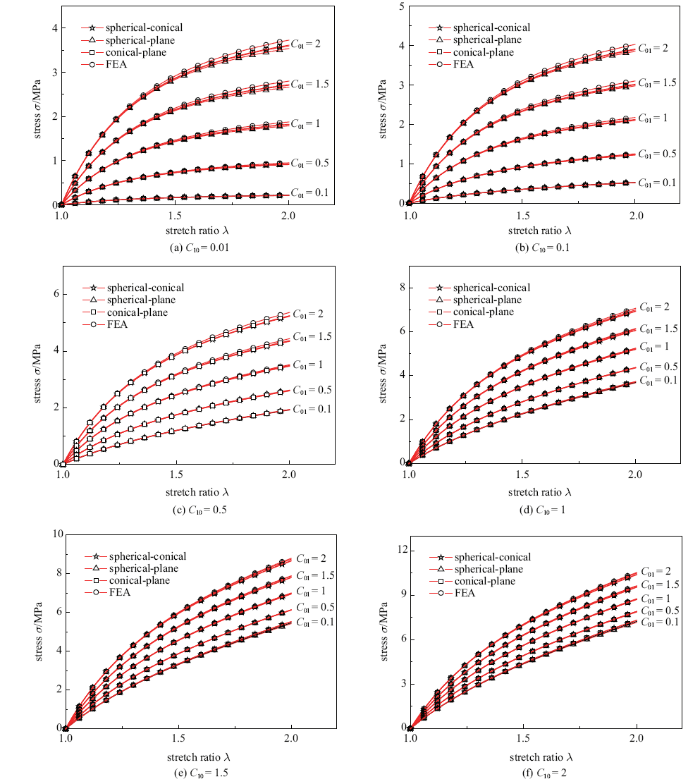Fig.4   Comparisons of predicted stress-stretch ratio curves and the FEA results

## 3 试验方法与验证

### 图5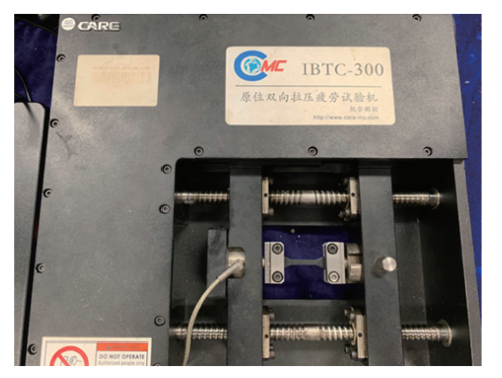Fig.5   Uniaxial tension test equipment

### 图6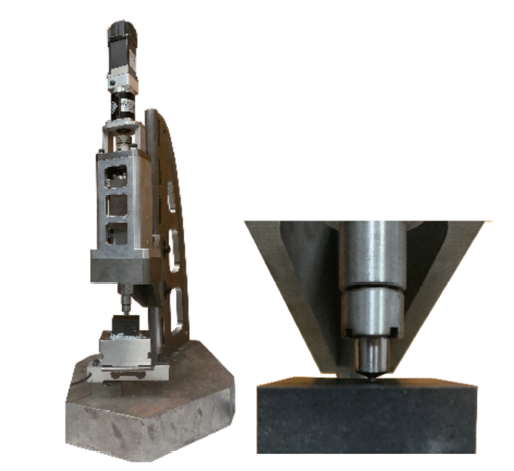Fig.6   Indentation test equipment

### 3.2 双压试验方法与试验比对

$\begin{equation} \label{eq17} \left. {\begin{array}{l} C_{S}=k_0 (k_1 C_{10} +k_2 C_{01} )|_{spherical}\\ C_{F} =k_0 (k_1 C_{10} +k_2 C_{01} )|_{flat} \\ C_{C}=k_0 (k_1 C_{10} +k_2 C_{01} )|_{conical}\\ \end{array}} \right\} \end{equation}$

$\begin{equation} \label{eq18} \left. {\begin{array}{l} \lambda =\dfrac{l+l_0 }{l_0 } \\ \sigma =\dfrac{P}{A} \\ \end{array}} \right\} \end{equation}$

### 图7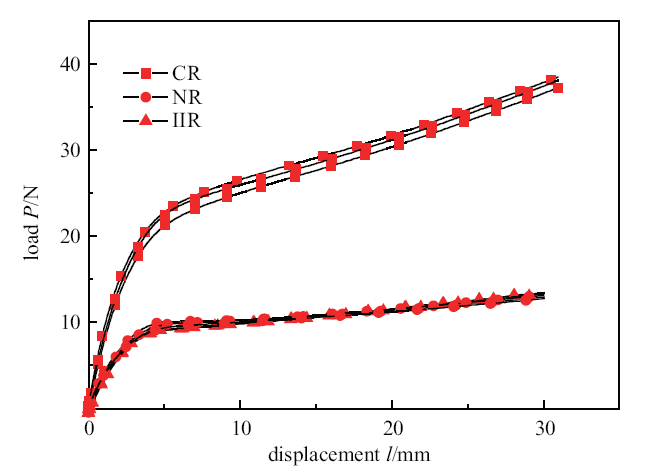### 图8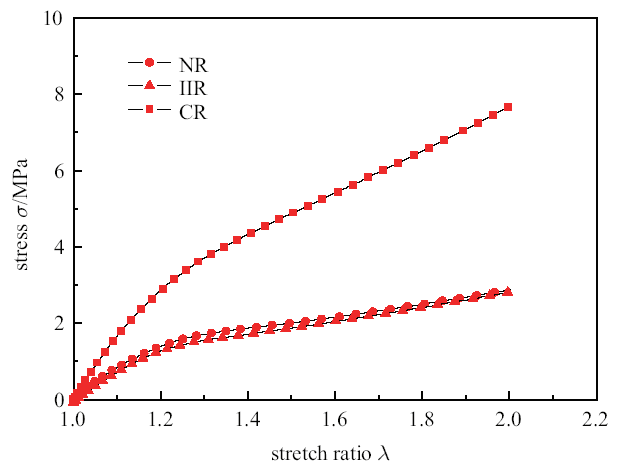Fig.8   Uniaxial stress-stretch ratio curve

### 图9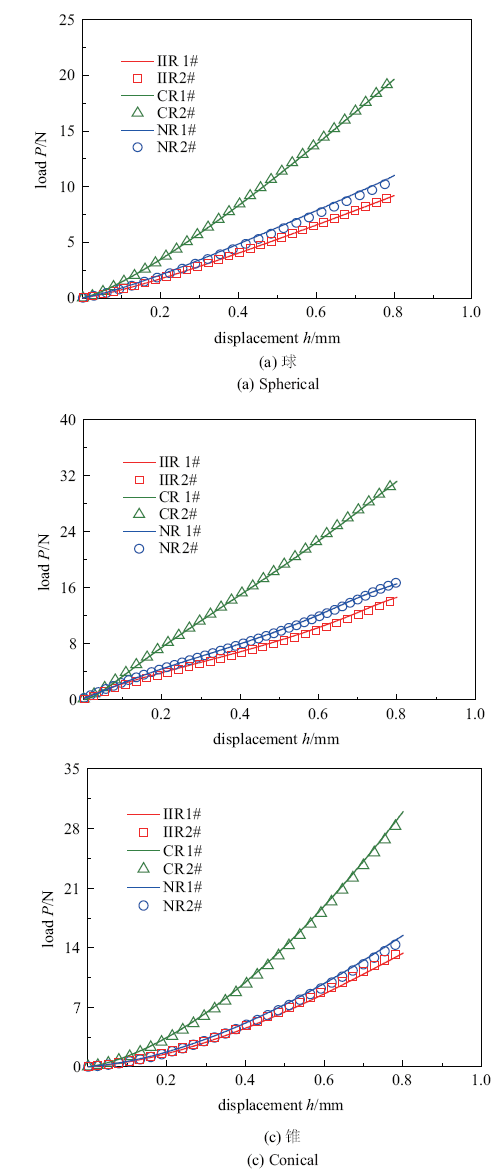### 图10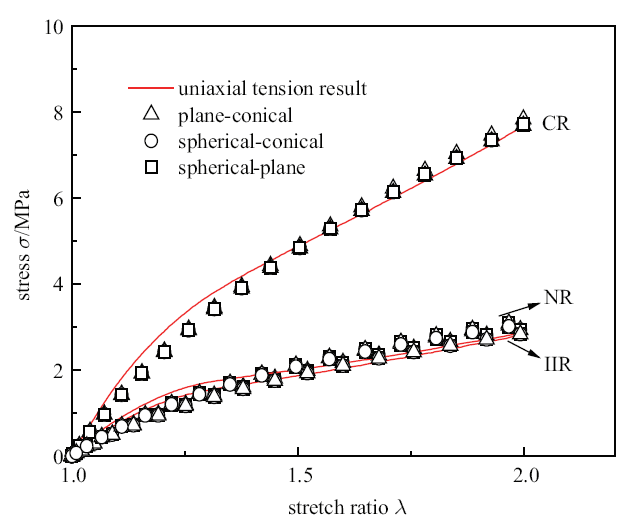Fig.10   Comparison between predicted stress-stretch ratio curve with the uniaxial tensile results

## 4 结论

(1)基于能量密度等效原理, 提出了球、平面、锥3类压头独立压入条件下, 描述载荷、深度、Mooney-Rivlin关系参数、几何尺寸之间关系的压入模型SHIM, SHIM正向预测的$P$/$P^*-h$/$D$压入曲线与FEA分析曲线之间密切吻合; 球、平面、锥压头两两组合压入下SHIM反向预测的3组本构关系曲线之间及与FEA条件本构关系曲线之间均密切吻合.

(2)提出了由球、平面、锥单压头两两组合压入下及具有不同半锥角的双锥压头压入下获取Mooney-Rivlin模型参数的双压试验方法.

(3)对3种橡胶材料分别进行单轴拉伸试验及球、平面、锥压入试验, 结果表明, 通过双压试验方法预测得到的本构关系曲线均与单轴拉伸试验结果具有良好的一致性.

/

 〈〉